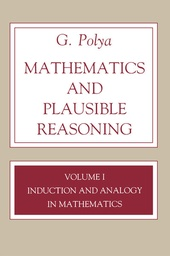# Mathematics and Plausible Reasoning, Volume 1

Induction and Analogy in Mathematics

Princeton University Press
• 1. Auflage
• |
• erschienen am 1. September 2020
• |
• 296 Seiten

 E-Book | PDF mit Adobe-DRM | Systemvoraussetzungen
978-0-691-21830-4 (ISBN)

A guide to the practical art of plausible reasoning, this book has relevance in every field of intellectual activity. Professor Polya, a world-famous mathematician from Stanford University, uses mathematics to show how hunches and guesses play an important part in even the most rigorously deductive science. He explains how solutions to problems can be guessed at; good guessing is often more important than rigorous deduction in finding correct solutions. Vol. I, on Induction and Analogy in Mathematics, covers a wide variety of mathematical problems, revealing the trains of thought that lead to solutions, pointing out false bypaths, discussing techniques of searching for proofs. Problems and examples challenge curiosity, judgment, and power of invention.

 Reihe: Sprache: Englisch Verlagsort: Princeton | USA Zielgruppe: Für Beruf und Forschung Editions-Typ: Digitale Ausgabe Produkt-Hinweis: Fixed format Dateigröße: 12,49 MB Schlagworte: ISBN-13: 978-0-691-21830-4 (9780691218304)weitere Ausgaben werden ermittelt
G. Polya
• Cover Page
• Half-title page
• Title page
• Preface
• Contents
• Chapter I: Induction
• 1. Experience and belief
• 2. Suggestive contacts
• 3. Supporting contacts
• 4. The inductive attitude
• Examples and Comments on Chapter I.
• Chapter II: Generalization, Specialization, Analogy
• 1. Generalization, Specialization, Analogy, and Induction
• 2. Generalization
• 3. Specialization
• 4. Analogy
• 5. Generalization, Specialization, and Analogy
• 6. Discovery by analogy
• 7. Analogy and induction
• Examples and Comments on Chapter II
• Chapter III: Induction in Solid Geometry
• 1. Polyhedra
• 2. First supporting contacts
• 3. More supporting contacts
• 4. A severe test
• 5. Verifications and verifications
• 6. A very different case
• 7. Analogy
• 8. The partition of space
• 9. Modifying the problem
• 10. Generalization, specialization, analogy
• 11. An analogous problem
• 12. An array of analogous problems
• 13. Many problems may be easier than just one
• 14. A conjecture
• 15. Prediction and verification
• 16. Again and better
• 17. Induction suggests deduction
• the particular case suggests the general proof
• 18. More conjectures
• Examples and Comments on Chapter III
• Chapter IV: Induction in the Theory of Numbers
• 1. Right triangles in integers
• 2. Sums of squares
• 3. On the sum of four odd squares
• 4. Examining an example
• 5. Tabulating the observations
• 6. What is the rule?
• 7. On the nature of inductive discovery
• 8. On the nature of inductive evidence
• Examples and Comments on Chapter IV
• Chapter V: Miscellaneous Examples of Induction
• 1. Expansions
• 2. Approximations
• 3. Limits
• 4. Trying to disprove it
• 5. Trying to prove it
• 6. The role of the inductive phase
• Examples and Comments on Chapter V
• Chapter VI: A More General Statement
• 1. Euler
• 2. Euler's memoir
• 3. Transition to a more general viewpoint
• 4. Schematic outline of Euler's memoir
• Chapter VII: Mathematical Induction
• 1. The inductive phase
• 2. The demonstrative phase
• 3. Examining transitions
• 4. The technique of mathematical induction
• Examples and Comments on Chapter VII
• Chapter VIII: Maxima and Minima
• 1. Patterns
• 2. Examples
• 3. The pattern of the tangent level line
• 4. Examples
• 5. The pattern of partial variation
• 6. The theorem of the arithmetic and geometric means and its first consequences
• Examples and Comments on Chapter VIII
• Chapter IX: Physical Mathematics
• 1. Optical interpretation
• 2. Mechanical interpretation
• 3. Reinterpretation
• 4. Jean Bernoulli's discovery of the Brachistochrone
• 5. Archimedes' discovery of the integral calculus
• Examples and Comments on Chapter IX
• Chapter X: The Isoperimetric Problem
• 1. Descartes' inductive reasons
• 2. Latent reasons
• 3. Physical reasons
• 4. Lord Rayleigh's inductive reasons
• 5. Deriving consequences
• 6. Verifying consequences
• 7. Very close
• 8. Three forms of the Isoperimetric Theorem
• 9. Applications and questions
• Examples and Comments on Chapter X
• Chapter XI: Further Kinds of Plausible Reasons
• 1. Conjectures and conjectures
• 2. Judging by a related case
• 3. Judging by the general case
• 4. Preferring the simpler conjecture
• 5. Background
• 6. Inexhaustible
• 7. Usual heuristic assumptions
• Examples and Comments on Chapter XI
• Final Remark
• Solutions to Problems
• Bibliography
Schweitzer Klassifikation
• Mathematik
•Allgemein
•Geschichte der Mathematik
• Mathematik
•Allgemein
•Philosophie der Mathematik
• Mathematik
•Geometrie
Newbooks Subjects & Qualifier
• Mathematik | Informatik
•Mathematik
•Mathematik Allgemein
•Geschichte der Mathematik
• Mathematik | Informatik
•Mathematik
•Mathematik Allgemein
•Philosophie der Mathematik
DNB DDC Sachgruppen
BIC Classifikation
• Mathematics & science
•Mathematics
•History of mathematics
• Mathematics & science
•Science: general issues
•Popular science
•Popular mathematics
BISAC ClassifikationDateiformat: PDF

Systemvoraussetzungen:

Computer (Windows; MacOS X; Linux): Installieren Sie bereits vor dem Download die kostenlose Software Adobe Digital Editions (siehe E-Book Hilfe).

Tablet/Smartphone (Android; iOS): Installieren Sie bereits vor dem Download die kostenlose App Adobe Digital Editions (siehe E-Book Hilfe).

E-Book-Reader: Bookeen, Kobo, Pocketbook, Sony, Tolino u.v.a.m. (nicht Kindle)

Das Dateiformat PDF zeigt auf jeder Hardware eine Buchseite stets identisch an. Daher ist eine PDF auch für ein komplexes Layout geeignet, wie es bei Lehr- und Fachbüchern verwendet wird (Bilder, Tabellen, Spalten, Fußnoten). Bei kleinen Displays von E-Readern oder Smartphones sind PDF leider eher nervig, weil zu viel Scrollen notwendig ist. Mit Adobe-DRM wird hier ein "harter" Kopierschutz verwendet. Wenn die notwendigen Voraussetzungen nicht vorliegen, können Sie das E-Book leider nicht öffnen. Daher müssen Sie bereits vor dem Download Ihre Lese-Hardware vorbereiten.

Bitte beachten Sie bei der Verwendung der Lese-Software Adobe Digital Editions: wir empfehlen Ihnen unbedingt nach Installation der Lese-Software diese mit Ihrer persönlichen Adobe-ID zu autorisieren!

Weitere Informationen finden Sie in unserer E-Book Hilfe.